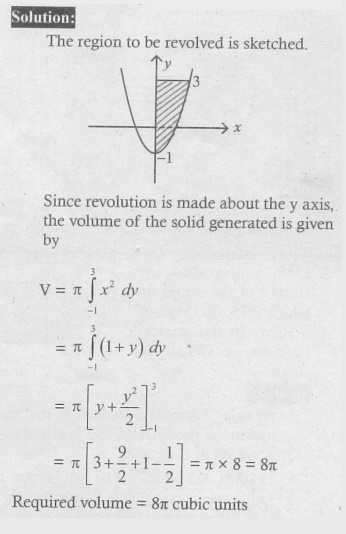Home | | Maths 12th Std | Exercise 9.9: Volume of a solid obtained by revolving area about an axis

# Exercise 9.9: Volume of a solid obtained by revolving area about an axis

Maths Book back answers and solution for Exercise questions - Mathematics : Applications of Integration: Volume of a solid obtained by revolving area about an axis - Exercise Problem Questions with Answer, Solution

EXERCISE 9.9

1. Find, by integration, the volume of the solid generated by revolving about the x-axis, the region enclosed by y = 2x2 , y = 0 and x = 1.2. Find, by integration, the volume of the solid generated by revolving about the x-axis, the region enclosed by y = eŌłÆ2x y = 0, x = 0 and x = 13. Find, by integration, the volume of the solid generated by revolving about the y-axis, the region enclosed by x2 = 1+ y and y = 3 .4. The region enclosed between the graphs of y = x and y = x2 is denoted by R, Find the volume generated when R is rotated through 360┬░ about x-axis.5. Find, by integration, the volume of the container which is in the shape of a right circular conical frustum as shown in the Fig 9.46.6. A watermelon has an ellipsoid shape which can be obtained by revolving an ellipse with major-axis 20 cm and minor-axis 10 cm about its major-axis. Find its volume using integration.1. 4ŽĆ / 5

2. ŽĆ/4 [1ŌłÆ eŌłÆ4]

3. 8 ŽĆ

4. 2ŽĆ/15

5. 14/3 ŽĆm3

6. 1000 ŽĆcm3

Tags : Problem Questions with Answer, Solution , 12th Maths : UNIT 9 : Applications of Integration
Study Material, Lecturing Notes, Assignment, Reference, Wiki description explanation, brief detail
12th Maths : UNIT 9 : Applications of Integration : Exercise 9.9: Volume of a solid obtained by revolving area about an axis | Problem Questions with Answer, Solution